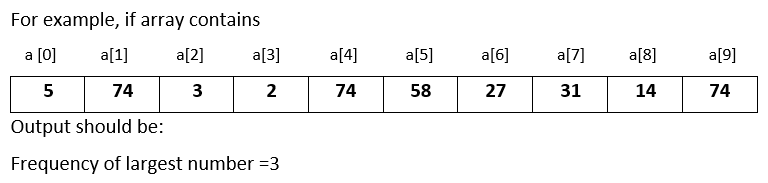# Input 10 numbers in 1d array and find the frequency of the largest number in Java

###### One Dimensional Array - Question 5

In this question, we will see how to input 10 numbers in a one dimensional integer array and find the frequency of the largest number in Java programming. To know more about one dimensional array click on the one dimensional array lesson.

Q5) Write a program in Java to input 10 numbers in a one dimensional integer array and find the frequency of the largest number.#### Program

``````import java.util.Scanner;

public class Q5
{
public static void main(String args[])
{
int a[]=new int, i,ln=0,c=0;
Scanner sc=new Scanner(System.in);

System.out.println("Enter 10 numbers");
for(i=0; i<10; i++)
{
a[i]=sc.nextInt();

if(i==0)
{
ln=a[i];
}
else if(a[i]>ln)
{
ln=a[i];
}
}

for(i=0; i<10; i++)
{
if(ln==a[i])
{
c++;
}
}

System.out.println("Frequency of the Largest Number = "+c);
}
}``````

#### Output

```Enter 10 numbers
5
74
3
2
74
58
27
31
15
74
Frequency of the Largest Number = 3  ```